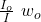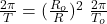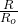## When a star like our sun runs out of nuclear fuel, it collapses to a white dwarf star the size of the Earth, but with the same mass the star

Question

When a star like our sun runs out of nuclear fuel, it collapses to a white dwarf star the size of the Earth, but with the same mass the star had before the collapse. If a star initially rotates once every 25 days, like our sun, what will its rotation rate be after it becomes a dwarf

in progress 0
2 months 2021-07-29T22:31:34+00:00 1 Answers 0 views 0

T = 1.8 10² s

Explanation:

This is an exercise of conservation of angular momentum,

Initial instant. Before collapsing

L₀ = I₀ w₀

Final moment. After the collapse

L_f = I w

as the system is isolated, the moment is conserved

L₀ = L_f

I₀ w₀ = I w

w =indicates that the rotation period is 25 day, let’s reduce to the SI system

T = 25 day (24 h / 1 day) (3600 s / 1 h) = 2.16 10⁶ s

Angular velocity and period are related

w = 2π/ T

The moment of inertia of a sphere is

I = 2/5 M R ²

in this case the moment before and after the collapse is

I₀ = 2/5 M R₀²

I = 2.5 M R²

the radius of a star like the sun is

R₀ = 6.96 10⁸ m

R = 6.371 10⁶ m

we substitute

w =T = ()² T₀

let’s calculate

T = (6.371 10⁶ / 6.96 10⁸ )² 2.16 10⁶

T = 1.8 10² s